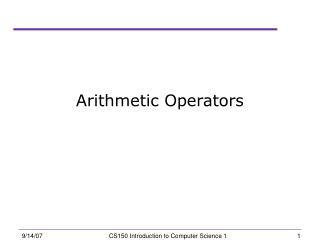Download PresentationArithmetic Operators

Arithmetic Operators - PowerPoint PPT Presentation

Arithmetic Operators. Today. Arithmetic Operators & Expressions sections 2.15 & 3.2 Computation Precedence Algebra vs C++ Exponents. Assigning float s to int s. Look at the following situation. int intVariable; intVariable = 42.7; cout << intVariable;I am the owner, or an agent authorized to act on behalf of the owner, of the copyrighted work described.
Download PresentationArithmetic Operators

Download Policy: Content on the Website is provided to you AS IS for your information and personal use and may not be sold / licensed / shared on other websites without getting consent from its author.While downloading, if for some reason you are not able to download a presentation, the publisher may have deleted the file from their server.

- - - - - - - - - - - - - - - - - - - - - - - - - - E N D - - - - - - - - - - - - - - - - - - - - - - - - - -
Presentation Transcript
1. Arithmetic Operators CS150 Introduction to Computer Science 1

2. Today • Arithmetic Operators & Expressions • sections 2.15 & 3.2 • Computation • Precedence • Algebra vs C++ • Exponents CS150 Introduction to Computer Science 1

3. Assigning floats to ints • Look at the following situation. int intVariable; intVariable = 42.7; cout << intVariable; • What do you think is the output? CS150 Introduction to Computer Science 1

4. Assigning floats to ints • What is the output here? int intVariable; double doubleVariable 78.9; intVariable = doubleVariable; cout << intVariable; CS150 Introduction to Computer Science 1

5. Arithmetic Operators • Operators allow us to manipulate data • Unary:operatoroperand • Binary: operandoperatoroperand (right hand side) (left hand side) CS150 Introduction to Computer Science 1

7. Division • grade = 100 / 40; grade is 2 • If both operands of the division operator are integers, then integer division is performed. • the data type of grade is not considered, why? • We say the integer is truncated. Everything after the decimal point is dropped. No rounding. • grade = 100.0 / 40; • grade is 2.5 • What data type should grade be declared as? CS150 Introduction to Computer Science 1

8. Modulus • Modulus is the remainder after integer division • grade = 100 % 20; • grade = ? • grade = 100 % 30; • grade = ? • rem = x % n; • What are the possible values for rem? CS150 Introduction to Computer Science 1

9. Practice • Q.1. What value is assigned to x? • x = 8 + 3; • x = 8 - 3; • x = 8 * 3; • x = 8 % 3; • x = 8 / 3; CS150 Introduction to Computer Science 1

10. Mathematical Expressions • Complex mathematical expressions are created by using multiple operators and grouping symbols • expression: programming statement that has value • sum = 21 + 3; • number = 3; In these two examples, we assign the value of an expression to a variable expression CS150 Introduction to Computer Science 1

11. Examples • result = x; • result = 4 + result; • result = 15 / 3; • result = 22 * number; • result = a + b % c; • result = a + b + d / c – q + 42; • cout << “The value: “ << (sum / 2) << endl; CS150 Introduction to Computer Science 1

12. Operator Precedence • result = a + b + d; • result = 12 + 6 / 3; • result = ? • Rules on how to evaluate an arithmetic expression • arithmetic expressions are evaluated left to right • when there are two operators, do them in order of precedence CS150 Introduction to Computer Science 1

13. Operator Precedence If two operators have the same precedence, evaluate them from left to right as they appear in the expression CS150 Introduction to Computer Science 1

14. Q.2. Practice • 5 + 2 * 3 • 10 / 2 -1 • 3 + 12 * 2 - 3 • 4 + 17 % 3 + 9 • 6 - 2 * 9 / 3 * 4 - 9 CS150 Introduction to Computer Science 1

15. Summary • Today we have looked at: • Arithmetic Operators & Expressions • Next time we will: • Continue looking at mathematic operators • Completed section 2.15 & started on section 3.2 CS150 Introduction to Computer Science 1